# How to Average Filtered Cells/List in Excel?

You could have applied the average function to tables in Excel, but have you ever tried to use the average in filtered cells? When we apply the direct formula to the filtered cells, the result is an error. There is a special method for finding the average for filtered cells. This tutorial will help you understand how we can average filtered cells in Excel. We can complete this process just by using the simple formulas supported by Excel.

## Average Filtered Cells/List in Excel

Here we will first create the table, then insert the slicer and use the SUBTOTAL formula to complete the task. Let us see a straightforward process to see how we can average filtered cells or lists in Excel.

Step 1

Consider an Excel sheet containing data similar to the data shown in the image below.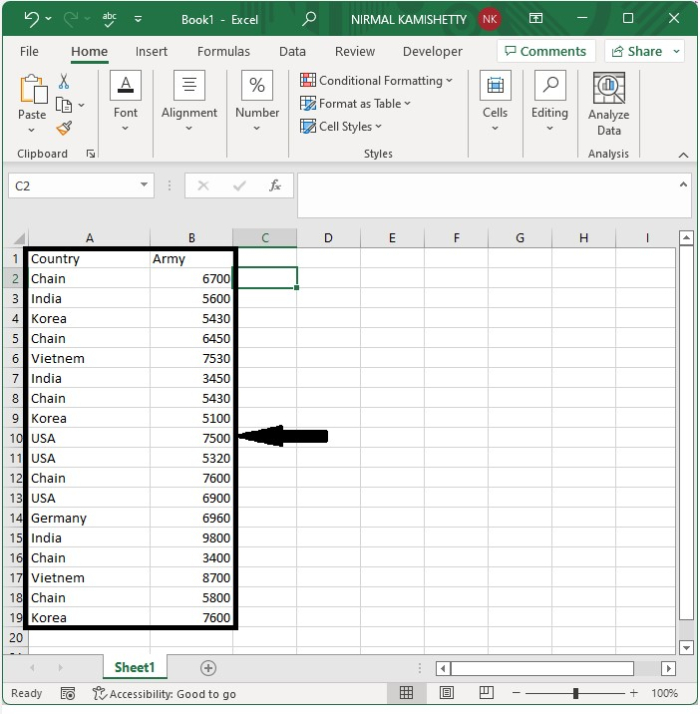As we all know, the filter can be applied to the tables. To create the table, select the data, then click on the table under Insert and click on OK to get the table as shown in the below image.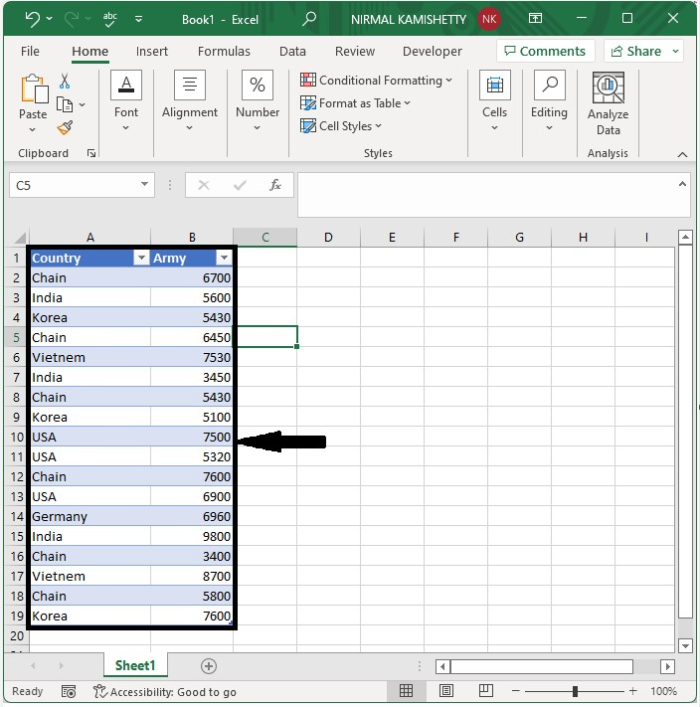Step 2

Now to apply the filter, click on "Insert" and select "Slicer" under the filter. The slicer will then be inserted into the sheet, as shown in the below image.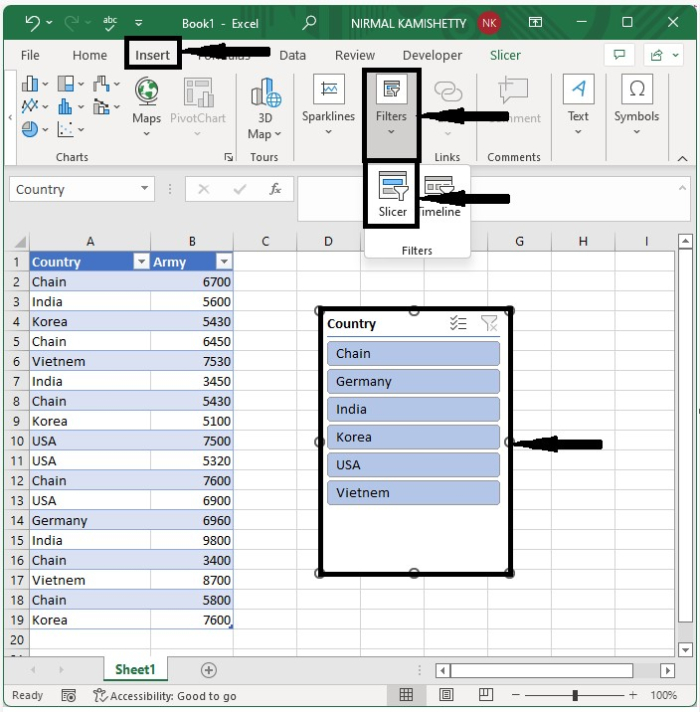Step 3

Then click on any button in the slicer to apply the filter, and the list will be filtered and the result will look like the below image. We will be filtering based on country, which is India.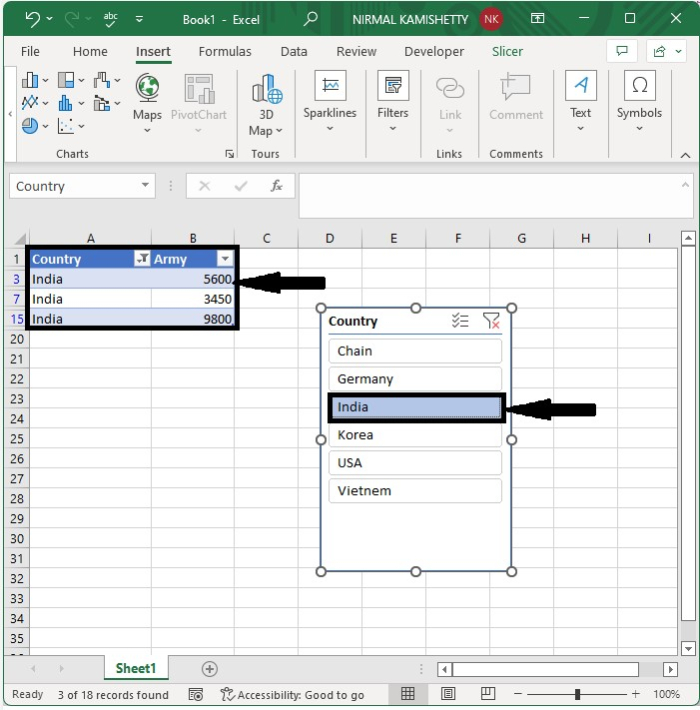Step 4

To get the average of filtered cells, click on an empty cell and enter the formula =SUBTOTAL(1,B2:B15) and press Enter. We will be using this formula because if we use the AVERAGE function, then the value will return an error, so we need to use the abovementioned formula.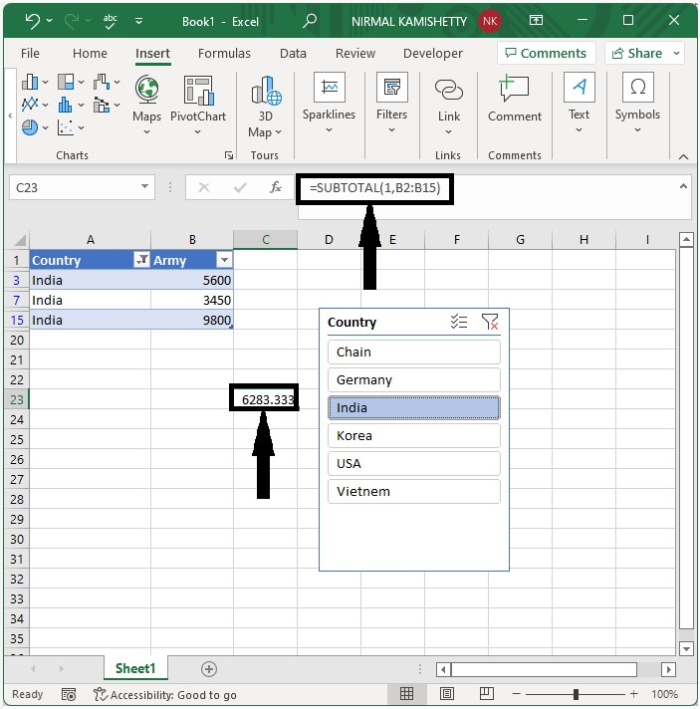## Conclusion

In this tutorial, we used a simple example to demonstrate how we can average filtered cells or lists in Excel.

Updated on: 12-Jan-2023

9K+ Views